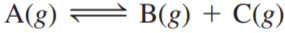×
Get Full Access to Chemistry: A Molecular Approach - 3 Edition - Chapter 14 - Problem 61e
Get Full Access to Chemistry: A Molecular Approach - 3 Edition - Chapter 14 - Problem 61e

×

# Consider the reaction: Find the equilibrium concentrationsISBN: 9780321809247 1

## Solution for problem 61E Chapter 14

Chemistry: A Molecular Approach | 3rd Edition

• Textbook Solutions
• 2901 Step-by-step solutions solved by professors and subject experts
• Get 24/7 help from StudySoup virtual teaching assistantsChemistry: A Molecular Approach | 3rd Edition

4 5 1 315 Reviews
10
2
Problem 61E

Consider the reaction:Find the equilibrium concentrations of A, B, and C for each value of Kc. Assume that the initial concentration of A in each case is 1.0 M and that the reaction mixture initially contains no products. Make any appropriate simplifying assumptions.

a. Kc = 1.0

b. Kc = 0.010

c. Kc = 1.0 x 10-5

Step-by-Step Solution:

Solution 61EStep 1The given chemical reactionEquilibrium expression

Step 2 of 18

Step 3 of 18

##### ISBN: 9780321809247

Chemistry: A Molecular Approach was written by and is associated to the ISBN: 9780321809247. This textbook survival guide was created for the textbook: Chemistry: A Molecular Approach, edition: 3. Since the solution to 61E from 14 chapter was answered, more than 434 students have viewed the full step-by-step answer. The full step-by-step solution to problem: 61E from chapter: 14 was answered by , our top Chemistry solution expert on 02/22/17, 04:35PM. The answer to “?Consider the reaction: Find the equilibrium concentrations of A, B, and C for each value of Kc. Assume that the initial concentration of A in each case is 1.0 M and that the reaction mixture initially contains no products. Make any appropriate simplifying assumptions.a. Kc = 1.0 b. Kc = 0.010 c. Kc = 1.0 x 10-5” is broken down into a number of easy to follow steps, and 57 words. This full solution covers the following key subjects: reaction, Equilibrium, assume, assumptions, Case. This expansive textbook survival guide covers 82 chapters, and 9454 solutions.

Unlock Textbook Solution